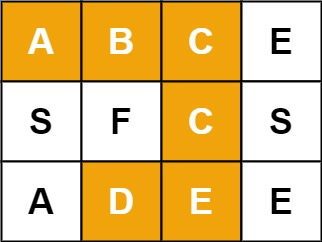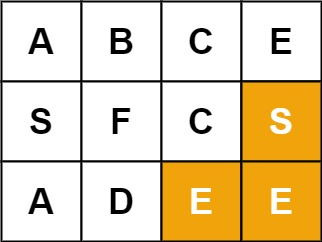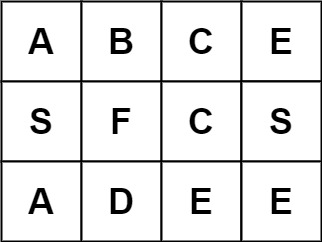# 79. 单词搜索

44

Given an `m x n` grid of characters `board` and a string `word`, return `true` if `word` exists in the grid.

The word can be constructed from letters of sequentially adjacent cells, where adjacent cells are horizontally or vertically neighboring. The same letter cell may not be used more than once.

Example 1:```Input: board = [["A","B","C","E"],["S","F","C","S"],["A","D","E","E"]], word = "ABCCED"
Output: true
```

Example 2:```Input: board = [["A","B","C","E"],["S","F","C","S"],["A","D","E","E"]], word = "SEE"
Output: true
```

Example 3:```Input: board = [["A","B","C","E"],["S","F","C","S"],["A","D","E","E"]], word = "ABCB"
Output: false
```

Constraints:

• `m == board.length`
• `n = board[i].length`
• `1 <= m, n <= 6`
• `1 <= word.length <= 15`
• `board` and `word` consists of only lowercase and uppercase English letters.

Follow up: Could you use search pruning to make your solution faster with a larger `board`?

#### 代码：

golang:

``````func exist(board [][]byte, word string) bool {
var maxLen = 0
var words = []byte(word)
var maxMatch = math.MinInt32
for i := 0; i < len(board); i++ {
for j := 0; j < len(board); j++ {
maxMatch = max(maxMatch, bfs(board, words, i, j, 0, maxLen))
if maxMatch == len(word) {
return true
}
}
}
return maxMatch == len(word)
}

var dests = [][]int{
[]int{-1, 0},
[]int{1, 0},
[]int{0, -1},
[]int{0, 1},
}

func bfs(board [][]byte, words []byte, i, j, idx int, maxLen int) int {
if i < 0 || j < 0 || i >= len(board) || j >= len(board) {
return maxLen
}

if idx == len(words) {
return maxLen
}

if board[i][j] != words[idx] {
return maxLen
}

maxLen++

// 四个方向继续探索
maxMatch := maxLen
var nowMaxLen = 0
for _, dest := range dests {

nowVal := board[i][j]
board[i][j] = '-'

nowMaxLen = maxLen
idx++
maxMatch = max(maxMatch, bfs(board, words, i+dest, j+dest, idx, maxLen))
idx--
maxLen = nowMaxLen
board[i][j] = nowVal
}

return maxMatch
}

func max(i, j int) int {
if i > j {
return i
}
return j
}
``````